Article citation information:

Mantič, M., Kuľka, J., Kopas, M., Faltinová, E., Hrabovský, L. Limit states of steel supporting structure for bridge cranes. Scientific Journal of Silesian University of Technology. Series Transport. 2020, 108, 141-158. ISSN: 0209-3324. DOI: https://doi.org/10.20858/sjsutst.2020.108.13.

Martin MANTIČ, Jozef KUĽKA, Melichar KOPAS, Eva FALTINOVÁ,
Leopold HRABOVSKÝ

LIMIT STATES OF STEEL SUPPORTING STRUCTURE FOR
BRIDGE CRANES

Summary. This paper describes a question of evaluation necessity of bridge cranes using the method of limit deformation state and oscillation damping. The solution was performed by means of theoretical analysis and an experimental verification at the selected bridge crane. The final result sounds that in the case of a correct strength computing of given bridge crane, it is not necessary to also check deformation and damping of oscillation as well.

Keywords: oscillation, damping, energy, experiment, deflection, time

1.    INTRODUCTION

The questions concerning vibrations of the bridge cranes are analysed in many professional works from various authors. For example, vibrations of the crane girder, which are occurring as a consequence of the crane travel on the crane track, are described by the authors in the works [1-3].

Scientific investigation of crane oscillations, together with the presentation of possibilities on how to eliminate these oscillations using a suitable form of the crane control strategy, is presented in [4-7].

Other methods, which are determined for the elimination of the crane oscillations by optimisation of the crane control components and mechanisms, are introduced in [8-10].

Different proposals of a dynamic model relating to the abovementioned problems are given in [11-14] and the corresponding experimental methods are in [15, 16].

Another interesting approach to the investigation of mechanical vibration, damage of transport machine parts and generation of failures is presented in [17-27].

Other experimental studies dealing with the mechanical properties of a layered beam, which is partially treated with a damping element based on a granular material, offers .

The author of , is focused on the assessment of vibration control performance using enhanced smart constrained layer damping treatment with edge elements. The non-linear dynamics of a crane is investigated in the contribution .

The commonly used or standard approach to the solution of a damping process represents the application of the logarithmic decrement, which enables estimation of the damping ratio from a time history of the oscillation process.

Analysis of this method and optimisation of the parameters during the processing and evaluation of the results is presented in [31, 32].

A new algorithm OMI (Optimization in Multiple Intervals), which is intended for computation of the logarithmic decrement concerning the exponentially damped harmonic oscillations, is described as well as compared with the classic computational methods in the publications [33, 34].

According to these articles, it is possible to say that the OMI algorithm is proved to be the best solution in the computation of the logarithmic decrement and the resonant frequency for high damping levels. Moreover, it is possible to take into consideration, a typical causal relation between the wear process and wear damage of constructional parts, which is introduced for example in .

The existing STN 27 0103  informs the design of steel crane structures according to two groups of limit states.

For the first group of limit states, which lead to the loss of loading capacity or the loss of position stability, the following criteria are central to the design of steel supporting structure:

·            strength and stability,

·            fatigue strength,

·            position stability.

For the second group of limit states, the following criteria are crucial:

·            static deformation (deflection, displacement, and twisting),

·            dynamic structure response (steel structure frequency, amplitude, and damping).

The second group of limit states is outlined in the Article X of the standard only in section 66, which states as follows: “Deformation and oscillation damping must not affect operational safety and must not interfere with proper crane function with regard to its work specifications”. In Annex VIII, the standard recommends how to approach the bridge crane evaluation.

To a great extent, the STN 27 0103  overlaps with the German DIN standard 15018 “Krane. Grundsätze für Stahlwerke. Berechnung“  in the calculation procedures. This DIN, however, does not specify checking for the limit state of deformation and damping.

This article lays out the theoretical analysis which consequently ties into the experimental analysis on a particular bridge crane.

2. OSCILLATION DAMPING OF A STEEL SUPPORTING STRUCTURE IN A BRIDGE CRANE

A degree of freedom in a flexible system is conceived as a number of independent coordinates which determine the location of all the system’s masses.

If the continuous distribution of the flexible elements is small in comparison with the masses distributed in the individual points, then the flexible element mass (m) can be downplayed and only the coordinates of the masses distributed in the points examined.

Let us propose that the resistance of the surroundings is directly proportional to the speed in the following relation:

Fresist  = – k.v , where k > 0,                                                   (1)

k – the damping coefficient (kg.s-1).

The resistance force counters the speed which is expressed by the minus sign in this formulation. If the acting restoring force is directly proportional to the displacement, the equation of motion is as follows: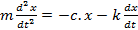,                                                      (2)

c – spring stiffness (N.m-1).

Once the substitution is applied: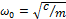and    2b = k/m,                                            (3)

where b is the damping coefficient and the ω0 constant is the natural angular frequency, that is, the angular frequency of the undamped harmonic oscillator.

After the adjustment, we get the following equation of motion:.                                                     (4)

The solution to the homogenous second order differential equation points to the following formulation:

x = eλt,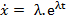,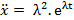.                                            (5)

After we apply the function to Equation 4, we get the following characteristic equation:,                                                           (6)

with this solution:.                                                     (7)

The two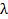values correspond to the general solution to Equation 4 in the linear combination: